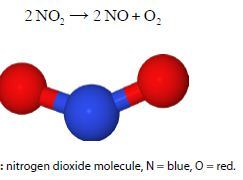# Explain Second-Order Chemical Reactions with Intregration

If the overall (or stoichiometric) reaction is two molecules of A reacting to give products 2A → P with rate constant k then for some mechanisms we may have a second-order reaction. An example of such a second-order chemical reactions are that of nitrogen dioxideNO2 is involved in important chemical reactions in polluted atmospheres and also in the industrial manufacture of nitric acid. Using the general symbol [A] rather than the specific one [NO2] we find the rate of loss of A is expressed by the second-order kinetics equation below.

d[A] / dt = – k [A]2

In order to obtain an equation which will give us the concentration of A at any time we need to integrate this equation from t = 0 when [A] = [A]0 to a general time t = t when [A] = [A]t. Carry out this definite integration for this second-order chemical reaction.

Sol

Using the method of “separation of variables” for the integration of the second-order kinetics equation.

d[A] / dt = – k [A]2

(1) Treat d[A] and dt as separate variables which follow the normal rules of algebra; then bring everything that involves [A] to the left hand side and everything that involves t or is a constant to the right hand side. (2) Now integrate both sides separately with the appropriate limits.

d[A] / [A]2 = – k dt

(3) An easy and foolproof way of integrating the left hand side of the equation is to write it as [A]-2 and then integrate as normal. You can use this “trick’ to integrate many equations which at first sight might look a bit difficult.